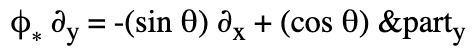# Ex. 19 Gauge Fields, Knots & Gravity: Is Rotation Correct?

• I
• ergospherical
In summary, the pushforward of a vector field created by a counterclockwise rotation by angle ##\theta## is equivalent to the rotation of the vector field.f

#### ergospherical

Wanted to check with you guys that I'm not going crazy...

Exercise 19: Let ##\phi : \mathbf{R}^2 \rightarrow \mathbf{R}^2## be a counterclockwise rotation by angle ##\theta##. Let ##\partial_x, \partial_y## be the coordinate vector fields on ##\mathbf{R}^2##. Show, at any point of ##\mathbf{R}^2##, that ##\phi_*\partial_x = (\cos{\theta})\partial_x - (\sin{\theta}) \partial_y## and also ##\phi_*\partial_y = (\sin{\theta})\partial_x + (\cos{\theta})\partial_y##

The effect of the pushforward is just a rotation of the vectors, so presumably one would instead expect ##\phi_* \partial_x = (\cos{\theta})\partial_x + (\sin{\theta})\partial_y##, right?

The rotation is ##(x,y) \mapsto \phi(x,y) = (x\cos{\theta} - y\sin{\theta}, \ x\sin{\theta} + y\cos{\theta})##. Let ##f \in C^{\infty}(\mathbf{R}^2)## be a test function, then the pushforward of ##\partial_x## is\begin{align*}
((\phi_* \partial_x)(f))(\phi(x),\phi(y)) &= (\partial_x(\phi^* f))(x,y) \\
&= (\partial_x (f \circ \phi))(x,y) \\
\end{align*}One can determine the ##x##-component of ##\phi_* \partial_x## by letting ##f=x##,\begin{align*}
((\phi_* \partial_x)(x))(\phi(x),\phi(y)) &= (\partial_x(x \circ \phi))(x,y) \\
&= (\partial_x(x\cos{\theta} - y\sin{\theta}))(x,y) \\
&= \cos{\theta}
\end{align*}Similarly, put ##f=y## to obtain ##((\phi_* \partial_x)(y))(\phi(x),\phi(y)) = \sin{\theta}##. Then\begin{align*}
\phi_* \partial_x = (\cos{\theta})\partial_x + (\sin{\theta})\partial_y
\end{align*}as before. I haven't misread something?

I agree with your conclusion. Alternatively, consider curve ##\gamma: t \mapsto (t,a)##, which has ##\partial_x## as its tangent vector. You will find that ##\phi \circ \gamma (t) = (t \cos\theta - a \sin\theta, t \sin\theta + a \cos\theta)##, which clearly has the tangent vector ##\cos\theta \partial_x + \sin\theta \partial_y##.

•ergospherical
Great, thanks!

•Orodruin
Is there an errata for the errata?